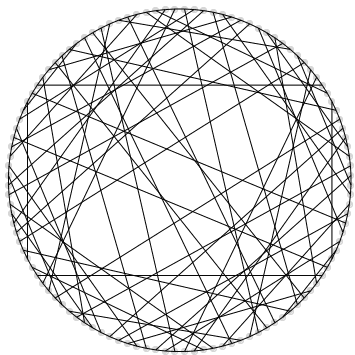Triangles: Interior and Exterior Angles - Maple Programming Help

# Online Help

###### All Products    Maple    MapleSim

Home : Support : Online Help : Math Apps : Algebra and Geometry : Geometry : MathApps/TrianglesInteriorAndExteriorAngles

Triangles: Interior and Exterior Angles

 Main Concept An interior angle of a polygon is formed by two sides of a polygon that share an endpoint. A shape has one internal angle per vertex. For a triangle, an interior angle is the angle between any two of the triangle's three sides. An exterior angle of a polygon is any of the angles formed by the intersection of one of the polygon's sides with a line extended from an adjacent side.   Properties: - The sum of the interior angles of a triangle is equal to 180 degrees. - The sum of the exterior angles of a triangle is equal to 360 degrees.

 Instructions The plot below shows a visual representation of the properties mentioned above, namely that the interior and exterior angles of the triangle add to 180 and 360 degrees respectively.   Choose either interior angles or exterior angles. Drag the red point to change the angles of the triangle and move the slider from left to right to see the proofs in motion. Interior Angles: The interior angles form a semi-circle (180 degrees). Exterior Angles: The exterior angles form a full circle (360 degrees).Slide to animate the proof:More MathApps